# Skew -Partitions of Balanced Size

The problem with recursive skew decomposition is that at least at first glance, you get exponential behavior. This would not occur if you were always able to find a decomposition in which each of A,B,C,D have at least n/c vertices for some c. Call this a skew partition of balanced size.

a) Can you find a skew partition of balanced in polynomial time, if one exists?

b) Will always looking for a balanced skew partition if possible lead to a polynomial size decomposition tree for perfect graphs (ie always use the skew partition which maximizes the size of the smallest set)

Answer: There exists a graph admitting no skew-partition of balanced size: take a clique and for some edges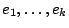of it add a vertices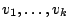s.t. each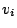has degree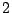and is adjacent to both ends of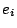.